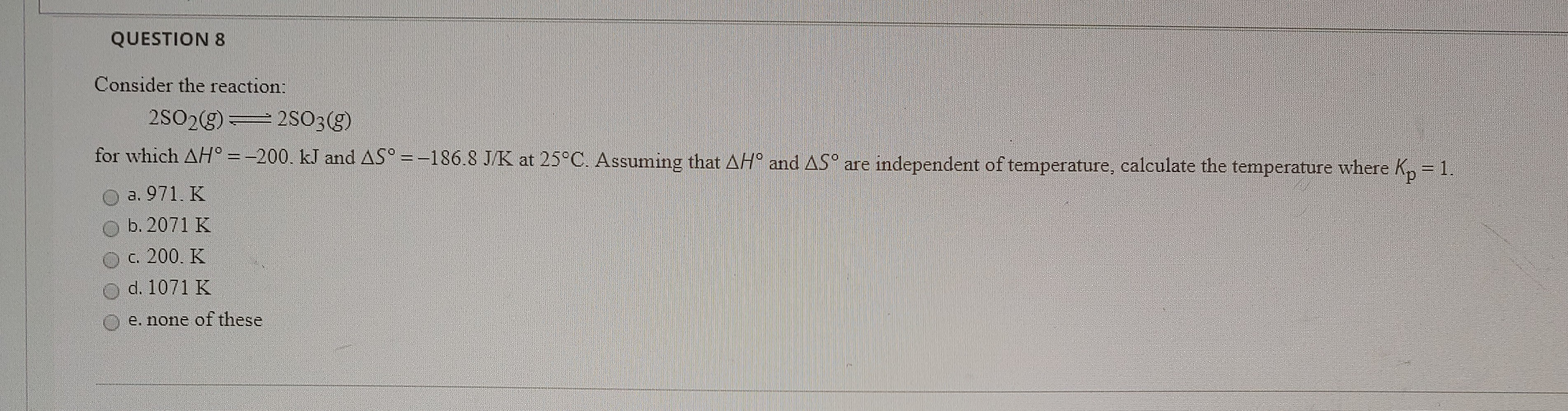# Consider the reaction: 2 SO2(g) ⇌ 2S SO3 (g) for which ΔH° = - 200 kJ and ΔS° = -186.8 J/K at 25°C. Assuming that ΔH° and ΔS° are independent of temperature, calculate the temperature where Kp = 1. a. 971 K b. 2071 K c. 200 K d. 1071 K e. none of these